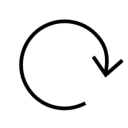◌◌◌ Interest
Simple And Compound Interest And Spreadsheet Intro
http://qindex.info/i.php?x=9774
○○○ start off ⪢⪢
Let's start off this lesson with an example of simple interest first and then of compound interest so that we can compare the two. #math
http://qindex.info/i.php?x=7026
---